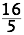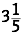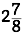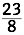# Breaking Up and Getting Back Together

Topic:
Fractions

## OBJECTIVE: To learn how to change improper fractions to mixed numbers and vice versa

IMPROPER FRACTIONS and MIXED NUMBERS are simply two different ways of expressing the same nonintegral number. In this lesson, you'll explore this idea and learn how to change one form to the other. Interact with the applet below to see the relationship between IMPROPER FRACTIONS and MIXED NUMBERS. Start by choosing a top-heavy fraction, a.k.a. improper fraction. Use the blue slider to choose the numerator and the red slider to choose the denominator. Make sure the numerator is larger than the denominator so that you'll get an improper fraction. Click Count Sectors to number and mark the parts. Use the green slider to dynamically show the conversion of your chosen improper fraction to a mixed number. Repeat as many times as needed to master the concept.

## HOW TO CHANGE AN IMPROPER FRACTION TO A MIXED NUMBER

EXAMPLE: What isas a mixed number? Divide the numerator by the denominator: 16 ÷ 5 = 3 remainder 1 The quotient (3) becomes the whole number and the remainder (1) becomes the numerator of the fraction next to the whole number; the denominator (5) doesn't change. So your answer is. Use the applet below for practice. Solve the problem first on a separate sheet of paper and then verify your answer. Repeat as many times as needed to master the concept.

## HOW TO CHANGE A MIXED NUMBER TO AN IMPROPER FRACTION

EXAMPLE: What isas an improper fraction? Multiply the denominator of the fraction to the whole number (8 x 2 = 16) and then add the numerator (16 + 7 = 23). This answer becomes the numerator of the improper fraction; the denominator stays the same:. Use the applet below for practice. Solve the problem first on a separate sheet of paper and then verify your answer. Repeat as many times as needed to master the concept.